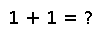# round(T::Type, x)

``````..  round([T,] x, [digits, [base]], [r::RoundingMode])

``round(x)`` rounds ``x`` to an integer value according to the default
rounding mode (see :func:`get_rounding`), returning a value of the same type as
``x``. By default (:obj:`RoundNearest`), this will round to the nearest
integer, with ties (fractional values of 0.5) being rounded to the even
integer.

.. doctest::

julia> round(1.7)
2.0

julia> round(1.5)
2.0

julia> round(2.5)
2.0

The optional :obj:`RoundingMode` argument will change how the number gets rounded.

``round(T, x, [r::RoundingMode])`` converts the result to type ``T``, throwing an
:exc:`InexactError` if the value is not representable.

``round(x, digits)`` rounds to the specified number of digits after the
decimal place (or before if negative). ``round(x, digits, base)`` rounds
using a base other than 10.

.. doctest::

julia> round(pi, 2)
3.14

julia> round(pi, 3, 2)
3.125

.. note::

Rounding to specified digits in bases other than 2 can be inexact when
operating on binary floating point numbers. For example, the ``Float64``
value represented by ``1.15`` is actually *less* than 1.15, yet will be
rounded to 1.2.

.. doctest::

julia> x = 1.15
1.15

julia> @sprintf "%.20f" x
"1.14999999999999991118"

julia> x < 115//100
true

julia> round(x, 1)
1.2``````

## Examples

Checking you are not a robot: